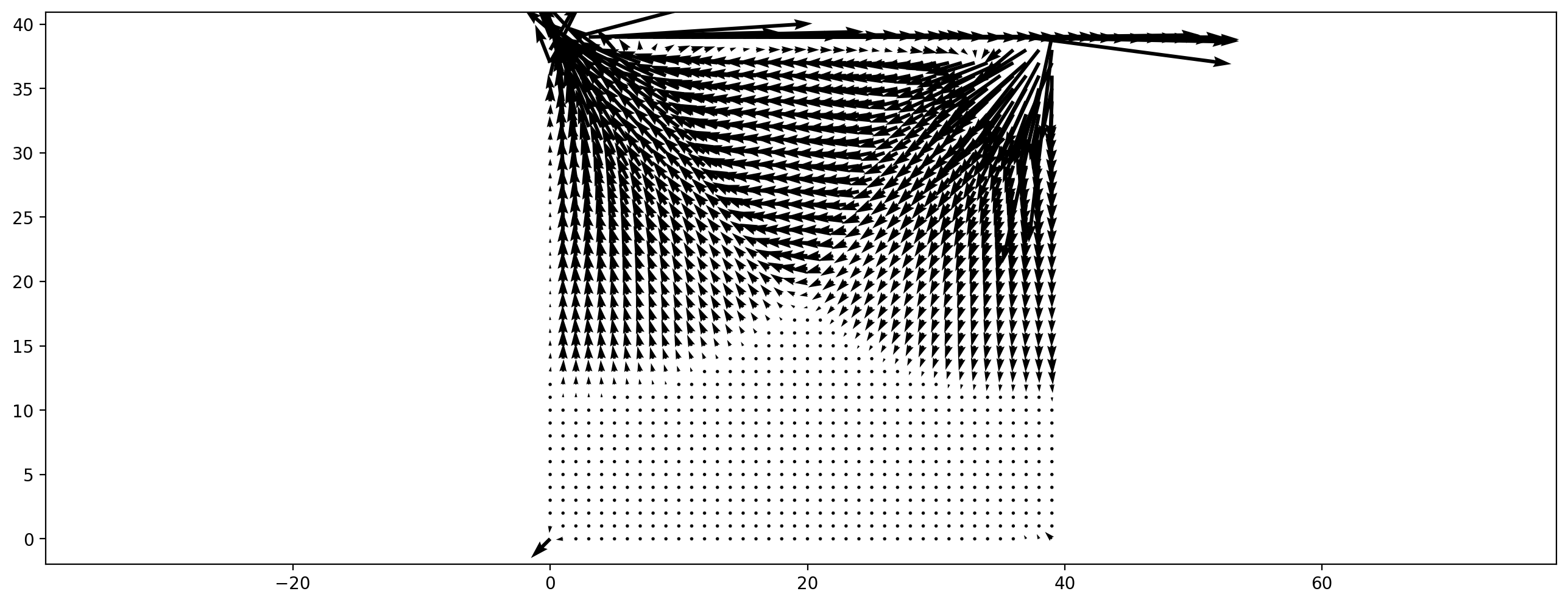In :
import pystencils as ps
from lbmpy.session import *
from lbmpy.moments import is_shear_moment, get_order


# Demo: Thermalized (Randomized) LBM¶

This demo notebook shows how to modify the LB collision operator to account for microscopic fluctuations. This technique is also called thermalized or randomized LBM. In these methods a random fluctuation is added to the equilibrium moments. In this simple example we implement a thermalized model by writing our own simple linear congruent random number generator, the draws indepedent random numbers on each cell. A seed is stored for each cell since all cells are processed in parallel.

## 1) Creating the method definition¶

We begin with a standard SRT method...

In :
random_number_symbols = sp.symbols("rand_:3")
method = create_lb_method(method='srt', relaxation_rate=1.8)
method

Out:
Moment Eq. Value Relaxation Rate
$1$ $\rho$ $1.8$
$x$ $u_{0}$ $1.8$
$y$ $u_{1}$ $1.8$
$x^{2}$ $\frac{\rho}{3} + u_{0}^{2}$ $1.8$
$y^{2}$ $\frac{\rho}{3} + u_{1}^{2}$ $1.8$
$x y$ $u_{0} u_{1}$ $1.8$
$x^{2} y$ $\frac{u_{1}}{3}$ $1.8$
$x y^{2}$ $\frac{u_{0}}{3}$ $1.8$
$x^{2} y^{2}$ $\frac{\rho}{9} + \frac{u_{0}^{2}}{3} + \frac{u_{1}^{2}}{3}$ $1.8$

...and modify its collision table. The create_lb_method_from_existing function provides a convenient way to do this. We pass a custom function that receives a row of the collision table and returns a modified version of it. Our modification rule adds symbols that stand for random numbers to some of the moments.

In :
def modification_func(moment, equilibrium, relaxation_rate):
if is_shear_moment(moment, dim=method.dim):
equilibrium += random_number_symbols * 0.001
elif get_order(moment) > 2:
equilibrium += random_number_symbols * 0.001
return moment, equilibrium, relaxation_rate

thermalized_method = create_lb_method_from_existing(method, modification_func)
thermalized_method.override_weights(method.weights)
thermalized_method

Out:
Moment Eq. Value Relaxation Rate
$1$ $\rho$ $1.8$
$x$ $u_{0}$ $1.8$
$y$ $u_{1}$ $1.8$
$x^{2}$ $0.001 rand_{0} + \frac{\rho}{3} + u_{0}^{2}$ $1.8$
$y^{2}$ $0.001 rand_{0} + \frac{\rho}{3} + u_{1}^{2}$ $1.8$
$x y$ $0.001 rand_{0} + u_{0} u_{1}$ $1.8$
$x^{2} y$ $0.001 rand_{1} + \frac{u_{1}}{3}$ $1.8$
$x y^{2}$ $0.001 rand_{1} + \frac{u_{0}}{3}$ $1.8$
$x^{2} y^{2}$ $0.001 rand_{1} + \frac{\rho}{9} + \frac{u_{0}^{2}}{3} + \frac{u_{1}^{2}}{3}$ $1.8$

## 2) Creating the kernel equations¶

Next we have to define rules how to compute the quantities $rand_0$ and $rand_1$. We do this using a linear congruent RNG on each cell. We pass in a seed array, and in each time step this seed array is updated with the new random numbers.

In :
dh = ps.create_data_handling(domain_size=(80, 80))

seed_type = np.uint32
max_seed_type = np.iinfo(seed_type).max

# Initialize the seed array
for b in dh.iterate():
random_field = np.random.randint(0, high=max_seed_type, dtype=seed_type, size=b['seed'].shape)
np.copyto(b['seed'], random_field)

linear_congruent_rng_eqs = [ps.Assignment(seedField(i), seedField(i) * 1664525 + 1013904223)
for i, _ in enumerate(random_number_symbols)]
floatEqs = [ps.Assignment(ps.TypedSymbol(s.name, np.float64), seedField(i) / max_seed_type)
for i, s in enumerate(random_number_symbols)]

rng_eqs = linear_congruent_rng_eqs + floatEqs + [ps.Assignment(debug_output.center, seedField(0) / max_seed_type)]
rng_eqs

Out:
$\displaystyle \left[ {{seed}_{(0,0)}^{0}} \leftarrow 1664525 {{seed}_{(0,0)}^{0}} + 1013904223, \ {{seed}_{(0,0)}^{1}} \leftarrow 1664525 {{seed}_{(0,0)}^{1}} + 1013904223, \ {{seed}_{(0,0)}^{2}} \leftarrow 1664525 {{seed}_{(0,0)}^{2}} + 1013904223, \ rand_{0} \leftarrow \frac{{{seed}_{(0,0)}^{0}}}{4294967295}, \ rand_{1} \leftarrow \frac{{{seed}_{(0,0)}^{1}}}{4294967295}, \ rand_{2} \leftarrow \frac{{{seed}_{(0,0)}^{2}}}{4294967295}, \ {{dbg}_{(0,0)}} \leftarrow \frac{{{seed}_{(0,0)}^{0}}}{4294967295}\right]$

These assignments are then added to the LB collision rule.

In :
collision_rule = create_lb_collision_rule(lb_method=thermalized_method)
collision_rule.subexpressions += rng_eqs


Finally, lets test our method by running a lid-driven-cavity with it.

In :
ldc = create_lid_driven_cavity(data_handling=dh, collision_rule=collision_rule, lid_velocity=0.05,
kernel_params={'rand_0': 0, 'rand_1': 0})

In :
#show_code(ldc.ast)

In :
ldc.run(100)

In :
plt.figure(dpi=200)
plt.vector_field(ldc.velocity[:, :]);In :
assert np.isfinite(dh.max('ldc_velocity'))